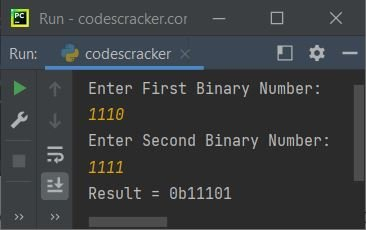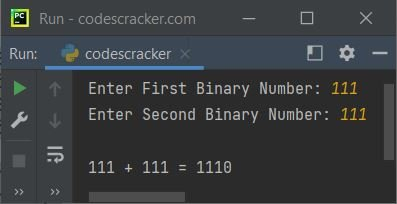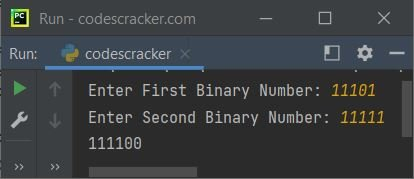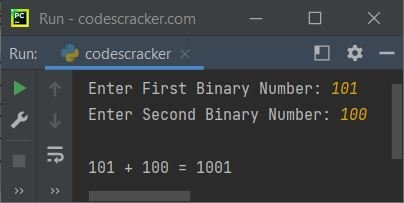# Python Program to Add Two Binary Numbers

In this article, you will learn and get code to add two binary numbers entered by the user using a Python program. Here is a list of programs for binary number addition:

• Add two binary numbers directly.
• Add two binary numbers using user-based code.

### How to Add Two Binary Numbers

Here are the rules for binary number addition:

```1 + 0 = 1
0 + 1 = 1
0 + 0 = 0
1 + 1 = 10 (0 and carry 1)
1 + 1 + 1 = 11 (1 and carry 1)```

For example

```  1 1 1 0 1
+ 1 1 1 1 1
-----------
1 1 1 1 0 0```

## Add two binary numbers directly

This program finds and prints the sum of two given binary numbers in a direct way. Here, direct way means that this program is created using int() and bin(), pre-defined functions of Python. Let's have a look at the program. I'll explain it later on.

```print("Enter First Binary Number: ")
nOne = int(input())
print("Enter Second Binary Number: ")
nTwo = int(input())

nOne = str(nOne)
nTwo = str(nTwo)
iSum = int(nOne, 2) + int(nTwo, 2)
bSum = bin(iSum)

print("Result = " + bSum)```

Here is its sample run:Now supply any two binary numbers, say 1110 as the first and 1111 as the second. Here is the sample run with exactly the same input:That is, 1110 + 1111 = 11101, or 0b1110 + 0b1111 = 0b11101.

Note: The int(num, 2) returns a decimal integer equivalent of the binary number stored in num. Here, 2 is the base of the number stored in num.

Therefore, the following statement:

`iSum = int(nOne, 2) + int(nTwo, 2)`

converts nOne and nTwo into their decimal integer equivalents and adds them. Its addition result gets initialized to the iSum variable. And using the bin() method, we've converted the decimal integer value into its equivalent binary number.

Note: The bin() function returns the binary equivalent string of an integer passed as its argument.

The dry run of the above program with user inputs 1110 and 1111 goes like this:

• When the user enters these two binary numbers, they get stored in nOne and nTwo, respectively. So nOne = 1110 and nTwo = 1111.
• Now, using nOne = str(nOne), nOne's value becomes a string value. That is, the type of nOne gets changed to a string type. Similar things happened with nTwo.
• Then using iSum = int(nOne, 2) + int(nTwo, 2)
int(nOne, 2) + int(nTwo, 2) or 14 + 15 or 29 gets initialized to iSum. As already mentioned, int(nOne, 2) returns the decimal integer equivalent of the value stored in nOne
• Finally, using bin(iSum), the value 29 gets converted into a binary equivalent string, which is 0b11101. 11101 is the binary equivalent of 29.
• Now just print the value of iSum as the addition result of two entered binary numbers.

#### Modified version of the previous program

This program is a modified version of the previous program with some better-looking output:

```print(end="Enter First Binary Number: ")
nOne = int(input())
print(end="Enter Second Binary Number: ")
nTwo = int(input())

nOne = str(nOne)
nTwo = str(nTwo)
iSum = int(nOne, 2) + int(nTwo, 2)
bSum = bin(iSum)

print("\n" + nOne + " + " + nTwo + " = " + bSum[2:])```

Here is its sample run with user input, with 111 as the first and 111 as the second binary numbers:Note: The bSum[2:] is used to print from the 2nd index. It is used to skip 0b of bSum. That is, 0 is at the 0th index, and b is at the 1st index.

## Add two binary numbers using user-defined code

This program is created with complete user-based code to add two binary numbers entered by the user. Because in this program, we've not used any type of pre-defined function:

```print(end="Enter First Binary Number: ")
nOne = int(input())
print(end="Enter Second Binary Number: ")
nTwo = int(input())

nOne = str(nOne)
nTwo = str(nTwo)
mLen = max(len(nOne), len(nTwo))
nOne = nOne.zfill(mLen)
nTwo = nTwo.zfill(mLen)
carry = 0

for i in range(mLen - 1, -1, -1):
re = carry
if nOne[i] == '1':
re = re+1
else:
re = re+0
if nTwo[i] == '1':
re = re+1
else:
re = re+0
if re%2==1:
else:
if re<2:
carry = 0
else:
carry = 1

if carry!=0:

Here is its sample run, with 11101 as the first binary number and 11111 as the second binary number:Note: The zfill() method is used to add zeros (0) at the beginning of the string. Its length is specified through its argument.

Note: The max() returns the maximum number from the numbers provided as its argument.

#### Modified version of the previous program

This program is again a modified version of the previous program. In this program, we've shortened the logical code to create a program that looks a little smaller than the previous one:

```print(end="Enter First Binary Number: ")
nOne = int(input())
print(end="Enter Second Binary Number: ")
nTwo = int(input())

nOne = str(nOne)
nTwo = str(nTwo)
mLen = max(len(nOne), len(nTwo))
nOne = nOne.zfill(mLen)
nTwo = nTwo.zfill(mLen)
carry = 0

for i in range(mLen - 1, -1, -1):
re = carry
re += 1 if nOne[i] == '1' else 0
re += 1 if nTwo[i] == '1' else 0
carry = 0 if re<2 else 1

if carry!=0:

print("\n" + nOne + " + " + nTwo + " = " + addRes)```

Here is its sample run with user input 101 as the first binary number and 100 as the second binary number:Python Online Test

« Previous Program Next Program »